# Introduction to Hashing

Hashing is a technique in Competitive Programming which is mostly used with string. In many cases, using hash can relieve you from doing other complex data structures or complicated dynamic programming optimization.

A drawback of hashing is that: you are not completely sure that your solution will work on all cases. If you are really really unlucky, your solution may fail one test-case and receive Wrong Answer. But the chance of failing is very low, and you can even lower that chance by using multiple hashes. So, in the scope of a programming contest, hashing is acceptable and can give you generous advantages. When applicable, hashing often helps you solve problems with a shorter time compared to the official solution.

It’s about 90% when we use Hash that we only care about lower-case English letters (not upper-case letters, digits nor other symbols). So in this post, I will assume that the string we hash contains only lower-case letters.

Hash function

There are several useful hash-functions. I will introduce in this post the most common one. It is common because it is simple and versatile. Let’s see:

Given a string s contains only lower-case English letters. As the length of s is s.length, we have:

s = s[0..(s.length-1)]

What we will do is to calculate an array, let’s name it ‘hash’, which has the same size as s, and hash[i] is a number that represents the substring s[0..i]. What I mean by “represents” is: when we compare 2 strings (or 2 substrings), we don’t need to loop through every character of the 2 strings (or substrings) to check if they are equal. Instead, we can compare their hash values. If their hash values are different, we are 100% sure that the 2 strings (or substrings) are different. If their hash values are equal, then the 2 strings or (substrings) are probably equal. 2 identical strings will have the same hash value. 2 different strings usually have different hash values, but there is very little chance that they can have the same hash value.

Back to the case of string s. Because s contains only lower-case letters (from a-z), so it can contain only 26 different characters. Let’s encode a equals to 1, b equals to 2, etc , z equals to 26. We will obtain array hash as follow:

hash = s

hash = hash +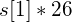hash = hash +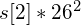hash = hash +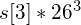hash[s.length – 1] = hash[s.length – 2] + s[s.length – 1]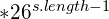For example, let s = “apple”. We have the array hash as: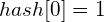(as ‘a’ = 1)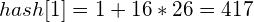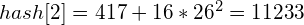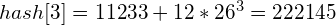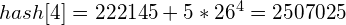Finally, hash = [1, 417, 11233, 222145, 2597025].

We can model this process on a table:

As we said above, hash[i] is a representation of s[0..i]. So for example, the hash value of ‘app’ is 11233, the hash value of ‘appl’ is 222145, etc.
But what is the hash value of ‘ppl’ (‘ppl’ is s[1..3])? It turns out that we can easily compute it using the hash array.

Let’s recall, normally, we will compute the hash value of ‘ppl’ as:
Hash value of ‘ppl’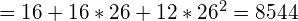. Or:

But wait, we already had the hash array of string ‘apple’. And the table of ‘apple’ and ‘ppl’ have something very similar. This is your exercise: let’s compare the 2 tables and try to obtain the hash value of ‘ppl’ using the table of ‘apple’!

And here is the result: On the table of ‘apple’, first we will remove the index 4 because the character ‘e’ has nothing to do with ‘ppl’. Then, we also remove the index 0, because ‘a’ is also not in ‘ppl’. Then the table becomes:

Ok, we have just the string ‘ppl’ on the table now. But we have to do one more thing: shift the string ‘ppl’ to the left. Because as in the table of ‘ppl’, its characters start from index position 0.

By shifting to the left 1 unit, we just have to divide the hash value for 26. That is divide 222144 by 26, and we will get 8544, which is the hash value of ‘ppl’.

Formally, we have:

hash value of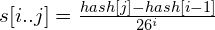This computation is done in just O(1), given we pre-calculated array hash and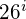for all i from 0 to s.length – 1.

MOD value

As you might have noticed, when we do as above, if the length of string s is longer, values in our array hash will be overflowed, even if we use long long type. That’s why we need a MOD value. That is, in each computation above, we always take the result mod the MOD value.

E.g.: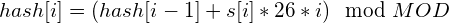, for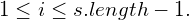hash value of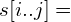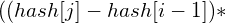modular-inverse())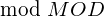.
(If you don’t know about modular-inverse, just search for “Fermat’s Little Theorem and Modular Inverse” on the internet.)

This will prevent our values from overflowing.

My code for Hash function is below.

struct HashFunction
{
string s;
ll *hash;
ll *modular_inverse;
ll *pexp;
ll p, HMOD;

ll mul_mod(ll x, ll y, ll MOD) {

if (MOD < (1<<31)) {
if (x > MOD)
x %= MOD;
if (y > MOD)
y %= MOD;

return (x * y) % MOD;
}

else {
ll res = 0, tmp = x%MOD;

while (y > 0) {
if (y&1)
res = (res + tmp) % MOD;

y >>= 1;
tmp = (tmp + tmp) % MOD;
}

return res;

}
}

ll pow_mod(ll x, ll exp, ll MOD) {
if (exp == 0)
return 1;

if (x > MOD)
x %= MOD;

ll half = pow_mod(x, exp >> 1, MOD);

if (exp & 1) {
return mul_mod(mul_mod(half, half, MOD), x, MOD);
}
else {
return mul_mod(half, half, MOD);
}
}

HashFunction(string s_, ll p_ = 26, ll HMOD_ = 1000000007) : s(s_), p(p_), HMOD(HMOD_) {

// compute hashing array and modular-inverse
hash = new ll[s.size()];
modular_inverse = new ll[s.size()];

ll seed = 1;
hash = s - '';
modular_inverse = 1;
pexp = 1;

for (int i = 1; i < s.size(); ++i) {
seed = (seed * p) % HMOD;

hash[i] =  (hash[i-1] + (s[i] - '')*seed) % HMOD;
modular_inverse[i] = pow_mod(seed, HMOD-2, HMOD);
pexp[i] = pexp[i-1] * p % MOD;
}

}

ll getHash(int i, int j) { // get hash of s[i..j]
if (i == 0)
return hash[j];
else
return ((hash[j] - hash[i-1] + HMOD) * modular_inverse[i]) % HMOD;

}

ll isEqualSubstring(int i, int j, int u, int v) { // compare s[i..j] and s[u..v]
if (i > u) {
swap(i, u);
swap(j, v);
}

ll hashFirst, hashSecond;

if (i == 0)
hashFirst = hash[j];
else
hashFirst = ((hash[j] - hash[i-1] + HMOD) % HMOD);

if (u == 0)
hashSecond = hash[v];
else
hashSecond = (hash[v] - hash[u-1] + HMOD) % HMOD;

return (hashFirst * pexp[u-i] % HMOD) == hashSecond ;
}

};

// Example:
// string s = "onetwothreetwoone";
// HashFunction hash(s);
// cout << hash.isEqualSubstring(0, 2, 14, 16) << endl;
// cout << hash.isEqualSubstring(0, 2, 13, 15) << endl;

Practice problems